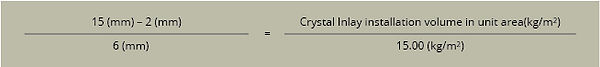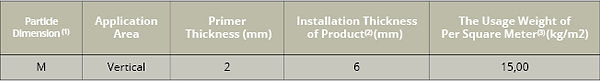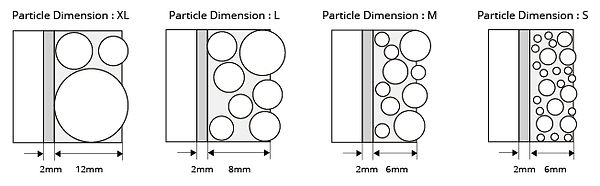Coverage

###### 3.Formula of the volume for installation under non-standard thickness condition

Formula:

(1) The”2” in the formula means the thickness of primer is 2mm.
1.The installation volume which is calculated by above formula is the practical unit volume of Crystal Inlay under non-standard thickness condition, in which primer is not included. In every pack, the primer is complete enough for usage.
2.If the practical installation thickness is much thicker than standard thickness, we would suggest before install Crystal Inlay, using concrete to fill up the standard thickness layer. This avoids added costs and wastage of the material.

Example:

For installation on a wall which reserves a depth of 15mm with the product CC W 300, with a total area of 3.75 square meters (1.5m×2.5m). How many kilograms of CC W 300 will be needed? What quantities of product are needed?

Step 1:The Practical Dimension of CC W 300 is “M”, which can be seen marked clearly on the drum.

Step 2:Check the Coverage table to find standard installation thickness and volume.

Step 3:According to the formula:

(1) You can find each particle dimension label on each package.

(2)The table above indicates the standard installation thickness of both the primer and product (CRYSTAL INLAY),  real installation thickness values may vary within the range of + 1mm.

(3)The usage weight of Crystal Inlay in this table is based on the standard thickness, therefore may vary during the actual installation, please understand the coverage may alter.

The usage weight does not include primer.

###### 2.Standard installation thickness on the vertical wall

(The thickness of installation depends on the average dimension of the particles size)Crystal Inlay practical installation volume per unit area =  (9-2)x15.00/6 = 17.5 (kg/m  )

Step 4:17.5 (kg/m2) x 4 (m2) = 70.00 kg Therefore, 70,00 kg of CC W 300 is needed.
Step 5:According to the Package Table there are three pack sizes available, small, medium and large.
The required quantities are as follows:
Large Package: 25 (kg/drum) x 2 drum = 50 kg →2 Drums should be needed
Middle Package: 5 (kg/drum) x 4 drum = 20 kg →4 Drum should be needed